# Canonical product

(diff) ← Older revision | Latest revision (diff) | Newer revision → (diff)

Weierstrass canonical product

An entire function with a given sequence of complex numbers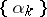as its zeros. Suppose that the zeros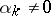are arranged in monotone increasing order of their moduli,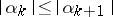, and have no limit point in the finite plane (a necessary condition), i.e.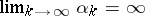. Then the canonical product has the form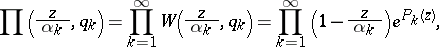where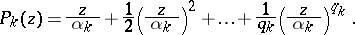The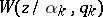are called the elementary factors of Weierstrass. The exponents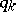are chosen so that the canonical product is absolutely and uniformly convergent on any compact set; for example, it suffices to take. If the sequence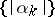has a finite exponent of convergence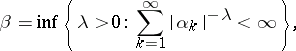then all thecan be chosen to be the same, starting, e.g. from the minimal requirement that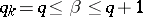; this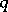is called the genus of the canonical product. If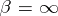, i.e. if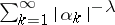diverges for any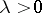, then one has a canonical product of infinite genus. The order of a canonical product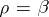(for the definition of the type of a canonical product, see ).

How to Cite This Entry:
Canonical product. Encyclopedia of Mathematics. URL: http://encyclopediaofmath.org/index.php?title=Canonical_product&oldid=19024
This article was adapted from an original article by E.D. Solomentsev (originator), which appeared in Encyclopedia of Mathematics - ISBN 1402006098. See original article